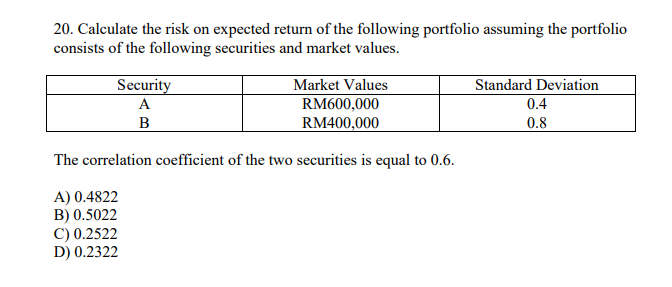Calculate the risk on expected return of the following portfolio assuming the portfolio consists of the following securities and market values

Calculate the risk on expected return of the following portfolio assuming the portfolio consists of the following securities and market values.

Market Values.   Standard Deviation

Security A RM600,000 0.4
Security B RM400,000 0.8

The correlation coefficient of the two securities is equal to 0.6.
A) 0.4822 B) 0.5022 C) 0.2522 D) 0.2322You know that big, long, ugly portfolio variance formula? This is just a plug 'n chug exercise.

1 Like